Thermodynamics and Propulsion
Subsections

# 19.4 Radiation Heat Transfer Between Black Surfaces of Arbitrary Geometry

In general, for any two objects in space, a given object 1 radiates to object 2, and to other places as well, as shown in Figure 19.10.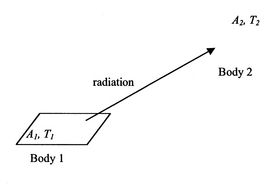We want a general expression for energy interchange between two surfaces at different temperatures. This is given by the radiation shape factor or view factor,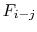. For the situation in Figure 19.11,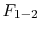= fraction of energy leaving 1 which reaches 2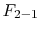= fraction of energy leaving 2 which reaches 1,are functions of geometry only

For body 1, we know that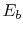is the emissive power of a black body, so the energy leaving body 1 is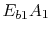. The energy leaving body 1 and arriving (and being absorbed) at body 2 is. The energy leaving body 2 and being absorbed at body 1 is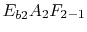. The net energy interchange from body 1 to body 2 is(19..4)

Suppose both surfaces are at the same temperature so there is no net heat exchange. If so,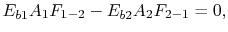but also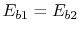. Thus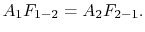Equation (19.4) is the shape factor reciprocity relation. The net heat exchange between the two surfaces is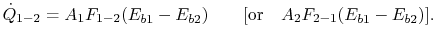## 19.4.1 Example: Concentric cylinders or concentric spheres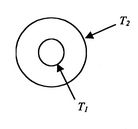The net heat transfer from surface 1 to surface 2 of Figure 19.12 is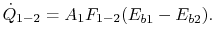We know that, i.e., that all of the energy emitted by 1 gets to 2. ThusThis can be used to find the net heat transfer from 2 to 1.View factors for other configurations can be found analytically or numerically. Shape factors are given in textbooks and reports (they are tabulated somewhat like Laplace transforms), and examples of the analytical forms and numerical values of shape factors for some basic engineering configurations are given in Figures 19.13 through 19.16, taken from the book by Incropera and DeWitt.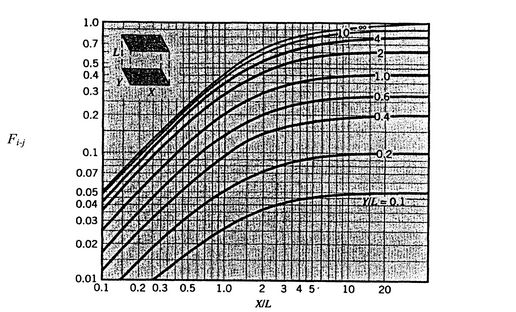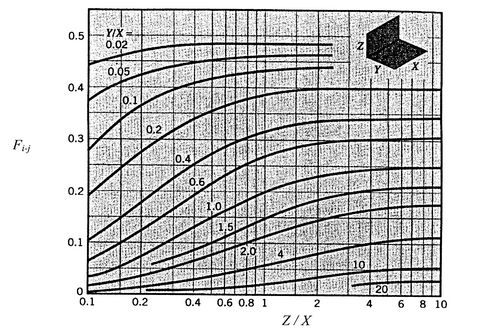UnifiedTP